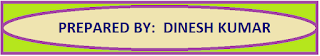### Common Errors in Secondary Mathematics

Common Errors Committed  by the  Students  in Secondary Mathematics   Errors  that students often make in doing secondary mathematics  during their practice and during the examinations  and their remedial measures are well explained here stp by step.  Some Common Errors in Mathematics

# ON CONIC SECTIONS

Smiley face which integrate all types of conic sections like Circle, Parabola, Ellipse and Hyperbola can be made with the help of mathematical equations and GeoGebra.

In this Blog we learn the following

1. Different types of conic sections like Circle, Parabola, Ellipse and Hyperbola.
2. Mathematical equations of different conic sections.
3. Art of drawing the conic sections by using GeoGebra.
4. Giving different colors to different shapes.
5. Integrating all conic sections with the art.
Conic sections are the sections of double napped cone. These are mainly of four types Circle, Parabola, Ellipse and Hyperbola. Each conic section have their mathematical equation as shown below in the figure.
We can visualize all these sections of cone with the help of GeoGebra. When we type the mathematical equations of different sections of cone at the input area of GeoGebra then we get the graphical shape.

REQUIREMENTS FOR MAKING THIS PROJECT

MATHEMATICAL EQUATION OF v  CIRCLE,

MATHEMATICAL EQUATION OF v  PARABOLA.

MATHEMATICAL EQUATION OF v  ELLIPSE

MATHEMATICAL EQUATION OF v  HYPERBOLA

USE OF GEOGEBRA

Following equations are used at the GeoGebra for making the Art Integrated Project on Conic Sections.

Equation of Circle (Face)                 x2 + y2 ≤ 16

Equation of Circle (Iris)                 (x - 2)2 + (Y - 1.5)2 ≤ 0.05

Equation of Circle (Iris)                 (x + 2)2 + (Y - 1.5)2 ≤ 0.05

Equation of Ellipse (Eye Ball)

Equation of Ellipse (Eye Ball)

Equation of Parabola  (Lips)            y + 2 = 0.5 x2 {-1.2 ≤ x ≤ 1.2}

Equation of Straight Line (Lips)     y = -1.3 {-1.2 ≤ x ≤ 1.2}

Equation of Parabola (Nose)           y + 0.7 = 4.5 x2 {- 0.5 ≤ x ≤ 0.5}

Equation of Hyperbola (Ear)

## USE OF GEOGEBRA

When we open GeoGebra app then we see following interface. In the figure given below we see two types of areas. one is graphical area where graphs in the form of shapes can be seen and the second is plan area where inputs can be given.

When we type the first equation of circle then in the graphical area we see the circle. This circle become the outer boundary of the Smiley face.

Now we type next two equations of circle. These equations make the Iris of the Eye Balls.

Now we type next two equations of Ellipse. These two equations make the two Eye Balls on the face.

Now we type an equation of Parabola. This equation make the Nose of the Smiley. For making the Lips we type one equation of Parabola and another equation of a straight line. These two equations made the Lips of the Smiley face.

For making the ear of the Smiley we write an equation of Hyperbola. This equation make the two ear of the smiley. In this way a complete Smiley face can be made at the GeoGebra.

In order to get clear smiley face then we have to remove the grid lines and the axis.

Smiley face can be made more attractive by changing the color of the Smiley. This project is completed now.

We can made different types of Smiley faces to make the activity more attractive for the students.

Here we use the mathematical equations of conic sections in an artistic way to make a Smiley face. This project integrate the mathematics with the help of Art. So it is called a Art Integrated Project on Mathematics.

Labeling the Smiley
Now we give labels to the Smiley
Face Boundary and Iris explain the Circle.
Both Eye Balls explain the Ellipse.
Both Ear represents the Hyperbola.
Nose and Lips represents the Parabola.

Smiley face can be made more attractive by giving different colors to the different Conic Sections.

Mathematical Equations of Conic sections which can be used directly on the GeoGebra for making the Smiley.

Equation of Circle:  x^(2)+y^(2)≤16

Equation of Circle: (x-2)^(2)+(y-1.5)^(2)≤0.05

Equation of Circle: (x+2)^(2)+(y-1.5)^(2)≤0.05

Equation of Ellipse: (((x-2)^(2))/(0.7^(2)))+(((y-1.9)^(2))/(1.2^(2)))=0.5

Equation of Ellipse:  (((x+2)^(2))/(0.7^(2)))+(((y-1.9)^(2))/(1.2^(2)))=0.5

Equation of Parabola: y+2=0.5 x^(2) {-1.2≤x≤1.2}

Equation of Straight Line:  y =-1.3 {-1.2≤x≤1.2}

Equation of Parabola:  y+0.7=4.5 x^(2) {-0.5≤x≤0.5}

Equation of Hyperbola:  ((x^(2))/(3^(2)))-((y^(2))/(2^(2)))=1 {-1.5≤y≤1.5}